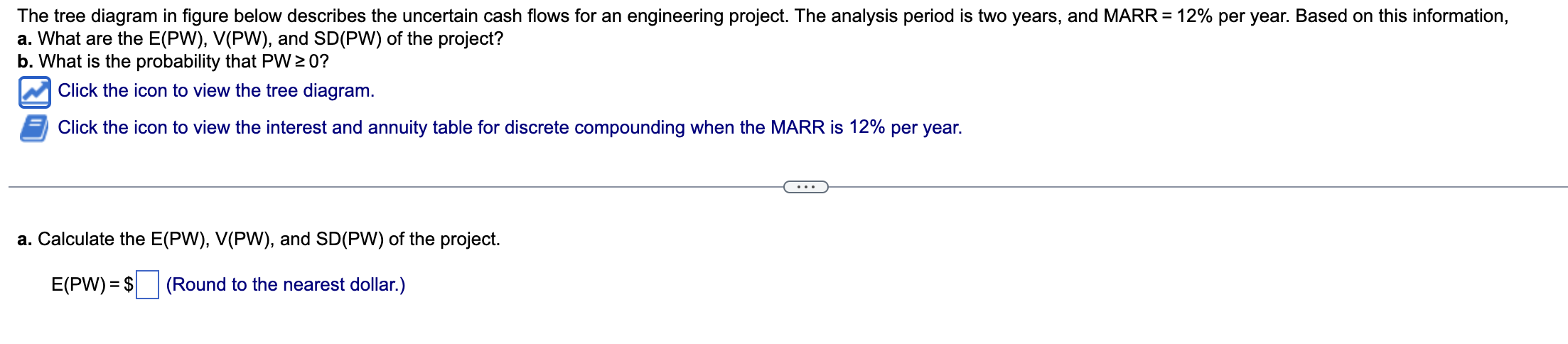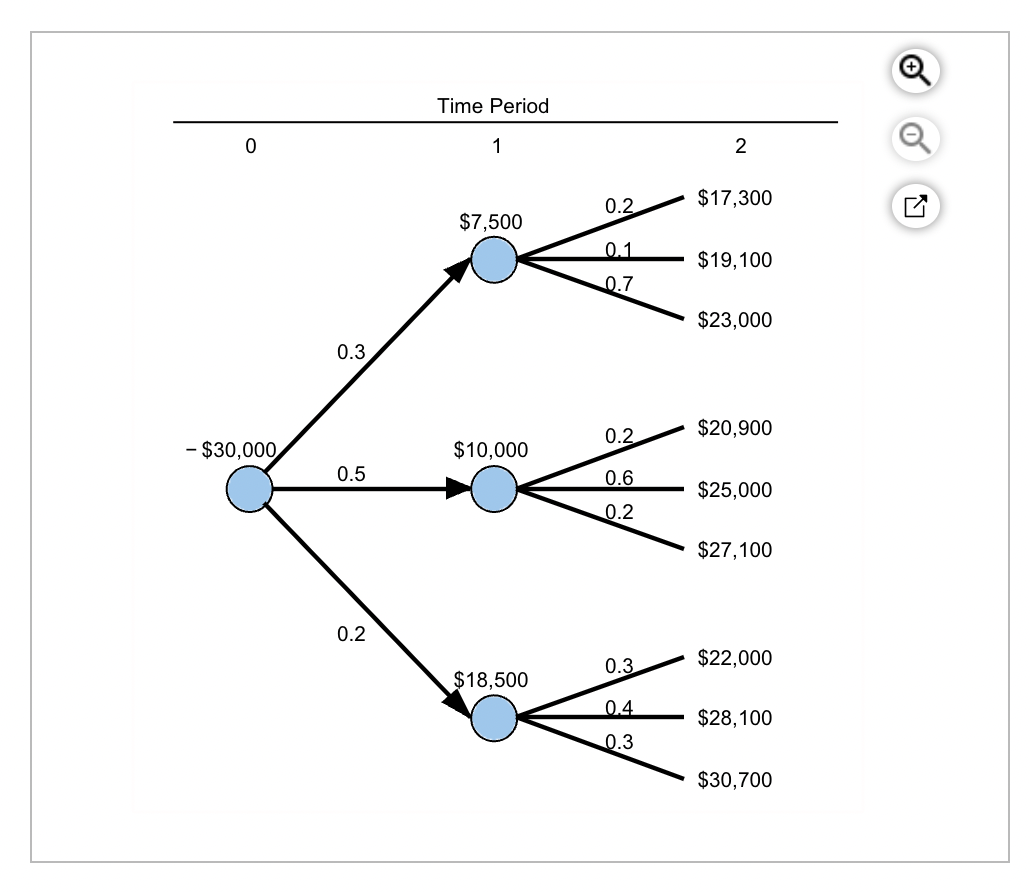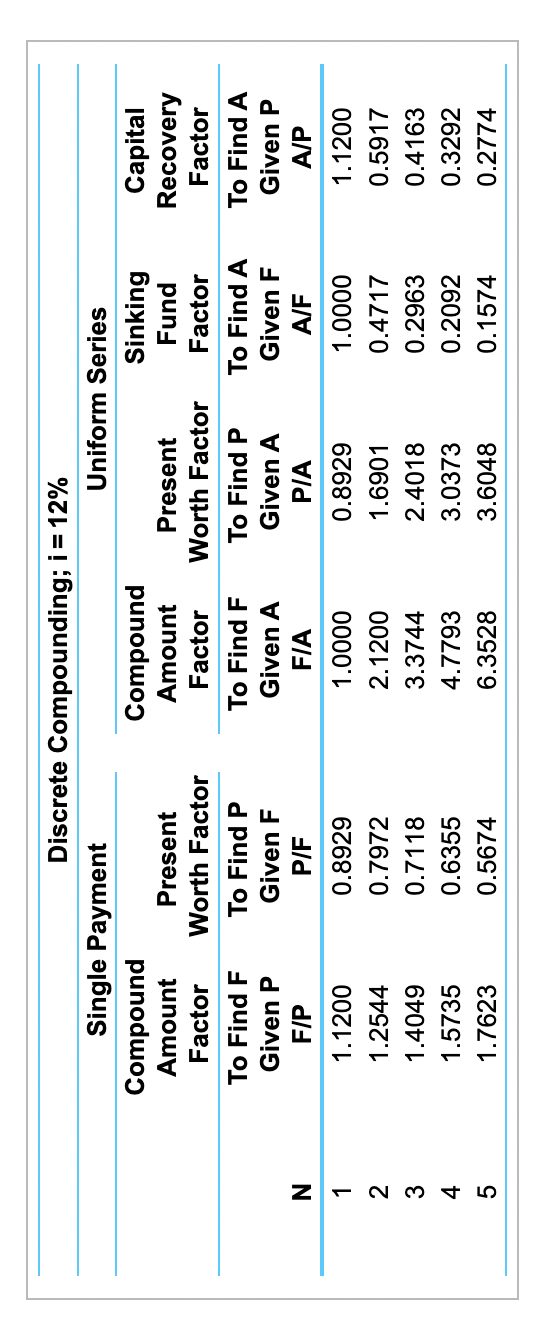Home / Expert Answers / Finance / the-tree-diagram-in-figure-below-describes-the-uncertain-cash-flows-for-an-engi-pa926

# (Solved): The tree diagram in figure below describes the uncertain cash flows for an engi ...???????The tree diagram in figure below describes the uncertain cash flows for an engineering project. The analysis period is two? years, and MARR?% per year. Based on this? information, a. What are the? E(PW), V(PW), and? SD(PW) of the? project? b. What is the probability that PW?0? LOADING... Click the icon to view the tree diagram. LOADING... Click the icon to view the interest and annuity table for discrete compounding when the MARR is ?% per year. Question content area bottom Part 1 a. Calculate the? E(PW), V(PW), and? SD(PW) of the project. ?E(PW)?\$ ?? enter your response here ?(Round to the nearest? dollar.)

The tree diagram in figure below describes the uncertain cash flows for an engineering project. The analysis period is two years, and MARR $$=12 \%$$ per year. Based on this information, a. What are the $$E(P W), V(P W)$$, and $$S D(P W)$$ of the project? b. What is the probability that $$P W \geq 0$$ ? Click the icon to view the tree diagram. Click the icon to view the interest and annuity table for discrete compounding when the MARR is $$12 \%$$ per year. a. Calculate the $$E(P W), V(P W)$$, and $$S D(P W)$$ of the project. $$E(P W)=\ \quad$$ (Round to the nearest dollar.) Time Period

We have an Answer from Expert